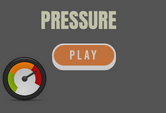# Pressure Game Trivia Online

This page features a Pressure Game Trivia Online. Pressure is a concept studied in physics and applied to many phenomena in real life. Atmospheric pressure is the weight the air around us exerts on us. Learn about pressure in this interactive game below. Click and start learning. 5th, 6th, 7th, 8th and 9th grades.

What is pressure? Simply put, pressure refers to the force exerted on an area. For example, pressure is applied when we nail a nail to a plank. Pressure is the force that is applied perpendicularly on the surface of an object for every unit area. This game will help you understand pressure better.

## Multiple Choice Questions On Pressure - Physics TestThe SI unit for pressure is Pa. What does Pa mean?

1. Pascal
2. Partial pressure
3. Parmesan
4. Pasteur

Pa is equal to…

1. Newton per meter
2. Newton per square meter
3. Newton per cubic meter
4. Newton per kilogram

What is the correct equation for calculating pressure?

1. Pressure = force*area
2. Pressure = force/area
3. Pressure = force+area
4. Pressure = force-area

What is atmospheric pressure?

1. The pressure exerted by air
2. The pressure exerted by water vapour
3. The pressure exerted by oxygen
4. The pressure exerted by carbon dioxide

Given that temperature remains constant, pressure is __________________ to volume.

1. Directly proportional
2. Unrelated
3. Equal
4. Inversely proportional

How much pressure does a 1600 N marble statue with a base of 2 m by 2 m exert on the ground?

1. 400 Pa
2. 600 Pa
3. 640 Pa
4. 800 Pa

Find the force that can apply a pressure of 700 Pa to an area of 1.4 square meters?

1. 770 N
2. 840 N
3. 910 N
4. 980 N

With constant volume, the pressure exerted on a gas is _______________ to its temperature.

1. Inversely proportional
2. Equal
3. Directly proportional
4. Unrelated

A 250 N crate on the floor has a base of 40 cm by 20 cm. How much pressure does it exert?

1. 3075 Pa
2. 3125 Pa
3. 3251.6 Pa
4. 3500 Pa

Pressure will be higher if force is subjected to a ______ area.

1. Smaller
2. Larger
3. Both A and B
4. There is no relation.

Find the force exerted by an object with a base of 2.5 m by 3 m if the resulting pressure is 375 Pa.

1. 2525.5 N
2. 2750.5 N
3. 2812.5 N
4. 3125.5 N

What do you use to measure atmospheric pressure?

1. Sphygmomanometer
2. Barometer
3. Piezometer
4. Manometer

Atmospheric pressure _________ as your altitude ________.

1. Decreases, increases
2. Increases, decreases
3. Stays the same, increases
4. Stays the same, decreases

What is the standard atmospheric pressure (atm) in kilopascals?

1. 100.325 kPa
2. 101.325 kPa
3. 101.335 kPa
4. 102.335 kPa

A pressure of 2500 Pa is experience when an object is subjected to a force of 3 kN. Calculate for its area.

1. 0.6 square meters
2. 1.2 square meters
3. 3.6 square meters
4. 6 square meters